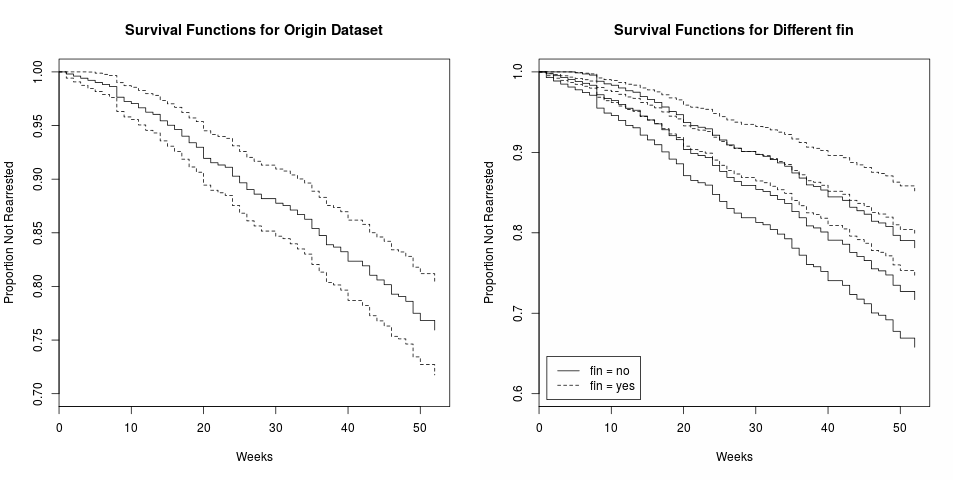# Cox Regression

##### Posted on Aug 17, 20170 Comments

Survival analysis examines and models the time it takes for events to occur. It focuses on the distribution of survival times. There are many well known methods for estimating unconditional survival distribution, and they examines the relationship between survival and one or more predictors, usually terms covariates in the survival-analysis literature. And Cox Proportional-Hazards regression model is one of the most widely used method of survival analysis.

## Terminology

• $T$ represents survival time;
• $P(t)=\Pr(T\le t)$ is the cumulative distribution of random variable $T$;
• $p(t)=dP(t)/dt$ is the probability density function;
• $S(t) = \Pr(T>t)=1-P(t)$ is the survival function.
• $h(t)$ is the hazard function, which assesses the instantaneous risk of demise at time $t$, conditional on survival to that time.
\begin{align*} h(t)&=\lim_{\Delta t\rightarrow 0}\frac{Pr[(t\le T<t+\Delta t)\mid T\ge t]}{\Delta t}\\ &=\lim_{\Delta t\rightarrow 0}\frac{S(t)-S(t+\Delta t)}{S(t)\Delta t}\\ &=-\frac{S'(t)}{S(t)}\\ &=\frac{f(t)}{1-F(t)} \end{align*}

Let’s take three kinds of hazard function, and they imply different distribution of survival times.

• If $h(t)=\nu$, where $\nu$ is a constant, it implies an exponential distribution of survival time.
• If $\log h(t)=\nu +\rho t$, it implies the Gompertz distribution of survival times.
• If $\log h(t)=\nu+\rho \log(t)$, it implies the Weibull distribution of survival times.

Censoring is a nearly universal feature of survival data, and there are three forms of censoring. Let me explain them in a medical study.

• right-censoring: some individuals may still be alive at the end of a medical study, or may drop out of the study for various reasons.
• left-censored: its initial time is unknown.
• interval-censoring: both right and left-censored.

## The Cox Proportional-Hazards Model

Survival analysis typically examines the relationship of the survival distribution to covariates. For example, a parametric model based on the exponential distribution may be written as,

$log h_i(t)=\alpha+\beta_1x_{i1}+\beta_2x_{i2}+\cdots+\beta_kx_{ik}$

where the constant $\alpha$ in the above model represents a kind of log-baseline hazard.

In contrast, the cox model leaves the baseline hazard function $\alpha(t)=log h_0(t)$ unspecified:

$log h_i(t)=\alpha(t)+\beta_1x_{i1}+\beta_2x_{i2}+\cdots+\beta_kx_{ik}$

The model is semi-parametric because while the baseline hazard can take any form, the covariates enter the model linearly. Consider two observations $i$ and $i’$,

$\eta_i=\beta_1x_{i1}+\beta_2x_{i2}+\cdots+\beta_kx_{ik}$

and

$\eta_{i'}=\beta_1x_{i'1}+\beta_2x_{i'2}+\cdots+\beta_kx_{i'k}$

The hazard ratio for these two observations,

$\frac{h_i(t)}{h_{i'}(t)}=\frac{exp(\eta_i)}{exp(\eta_{i'})}$

is independent of time $t$. Consequently, the Cox model is a proportional-hazards model.

## An Illustration

The details of dataset can refer to the references at the end of this post.Cox Proportional-Hazards Regression for Survival Data in R